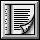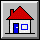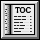5.5 Class NUMERIC`indexing`
```description: "Objects to which numerical operations are applicable";
note: "The model is that of a commutative ring."```
`deferred class interface`
`NUMERIC`
`feature -- Access`
```one: like Current
-- Neutral element for "*" and "/"
deferred
ensure
Result_exists: Result /= Void```
```zero: like Current
-- Neutral element for "+" and "-"
deferred
ensure
Result_exists: Result /= Void```
`feature -- Status report`
```divisible (other: like Current): BOOLEAN
-- May current object be divided by other?
require
other_exists: other /= Void
deferred
```
```exponentiable (other: NUMERIC): BOOLEAN
-- May current object be elevated to the power other?
require
other_exists: other /= Void
deferred```
`feature -- Basic operations`
```infix "+" (other: like Current): like Current
-- Sum with other (commutative).
require
other_exists: other /= Void
deferred
ensure
result_exists: Result /= Void;
commutative: equal (Result, other + Current)```
```infix "-" (other: like Current): like Current
-- Result of subtracting other
require
other_exists: other /= Void
deferred
ensure
result_exists: Result /= Void```
```infix "*" (other: like Current): like Current
-- Product by other
require
other_exists: other /= Void
deferred
ensure
result_exists: Result /= Void```
```infix "/" (other: like Current): like Current
-- Division by other
require
other_exists: other /= Void;
good_divisor: divisible (other)
deferred
ensure
result_exists: Result /= Void```
```infix "^" (other: NUMERIC): NUMERIC
-- Current object to the power other
require
other_exists: other /= Void;
good_exponent: exponentiable (other)
deferred
ensure
result_exists: Result /= Void```
```prefix "+": like Current
-- Unary plus
deferred
ensure
result_exists: Result /= Void```
```prefix "-": like Current
-- Unary minus
deferred
ensure
result_exists: Result /= Void```
`invariant`
```neutral_addition: equal (Current + zero, Current);
self_subtraction: equal (Current - Current, zero);
neutral_multiplication: equal (Current * one, Current);
self_division: divisible (Current) implies equal (Current / Current, one)```
`end`

 Copyright © 1995, Nonprofit International Consortium for Eiffel mailto:nice@atlanta.twr.com Last Updated: 26 October 1997